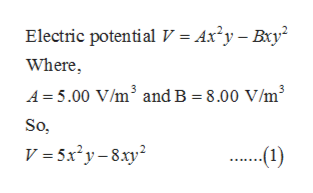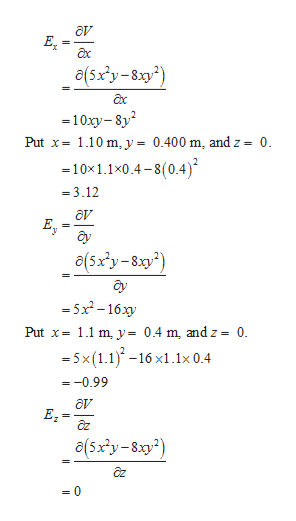# In a certain region of space the electric potential is given by V=+Ax^2y−Bxy^2, where A= 5.00 V/m^3 and B= 8.00 V/m^3.Part A Calculate the magnitude of the electric field at the point in the region that has coordinates x= 1.10 m, y= 0.400 m, and z= 0. Express your answer to three significant figures and include the appropriate units.Part B Calculate the direction angle of the electric field at the point in the region that has coordinates x= 1.10 m, y= 0.400 m, and z= 0. Express your answer in degrees.∘, measured counterclockwise from the positive x-axis in the xy plane

Question
41 views

In a certain region of space the electric potential is given by V=+Ax^2y−Bxy^2, where A= 5.00 V/m^3 and B= 8.00 V/m^3.

Part A
Calculate the magnitude of the electric field at the point in the region that has coordinates x= 1.10 m, y= 0.400 m, and z= 0.
Express your answer to three significant figures and include the appropriate units.

Part B
Calculate the direction angle of the electric field at the point in the region that has coordinates x= 1.10 m, y= 0.400 m, and z= 0.

∘, measured counterclockwise from the positive x-axis in the xy plane

check_circle

Step 1

Given,help_outlineImage TranscriptioncloseElectric potential v = Ax'y-Bxy Where A 5.00 V/m3 and B = 8.00 V/m3 So, V = 5x2y-8xy .1) fullscreen
Step 2

Part A: The magnitude of the electric field at the point in the region that has coordinates x = 1.1 m, y = 0.4 m, and z = 0.

Partially differentiate equation (1) with respect to x, y and z respectively:help_outlineImage TranscriptioncloseEL a(5xy-8xy2) =10xy-8y Put x1.10 m, y = 0.400 m, and z = 0. - 10x1.1x0.4-8(0.4) =3.12 ду a(5x'y-8xy2) 5x-16 Put x1.1 m, y= 0.4 m, and z = 0. -5x(1.1)16x.x 0.4 =-0.99 дv Ez a(5x'y-8xy2) =0 fullscreen
Step 3

Magnitude of the electric field:

...

### Want to see the full answer?

See Solution

#### Want to see this answer and more?

Solutions are written by subject experts who are available 24/7. Questions are typically answered within 1 hour.*

See Solution
*Response times may vary by subject and question.
Tagged in

### Electric Charges and Fields